$$~$$

After the niceViolin() function, here’s how to make nice scatter plots easily!

Let’s first load the demo data. This data set comes with base R (meaning you have it too and can directly type this command into your R console).

data("mtcars")
head(mtcars)
##                    mpg cyl disp  hp drat    wt  qsec vs am gear carb
## Mazda RX4         21.0   6  160 110 3.90 2.620 16.46  0  1    4    4
## Mazda RX4 Wag     21.0   6  160 110 3.90 2.875 17.02  0  1    4    4
## Datsun 710        22.8   4  108  93 3.85 2.320 18.61  1  1    4    1
## Hornet 4 Drive    21.4   6  258 110 3.08 3.215 19.44  1  0    3    1
## Hornet Sportabout 18.7   8  360 175 3.15 3.440 17.02  0  0    3    2
## Valiant           18.1   6  225 105 2.76 3.460 20.22  1  0    3    1

Source the function from my github:

source("https://raw.githubusercontent.com/RemPsyc/niceplots/master/niceScatterFunction.R")

$$~$$

### Make the basic plot

*Warning:* running the function below for the first time will install and load the following
Note: This will run many lines of code on your console and could take 5 minutes or more.
niceScatter(data = mtcars,
predictor = wt,
response = mpg)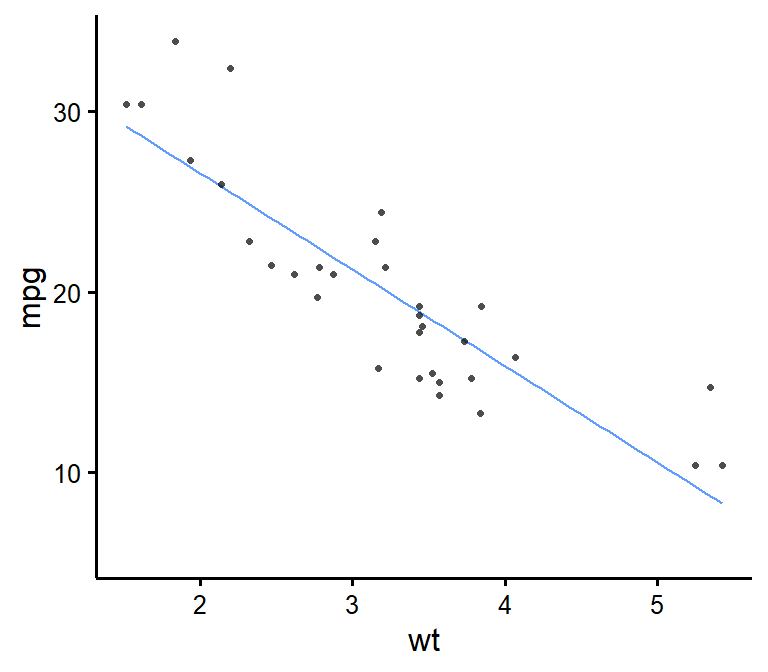### Save a high-resolution image file to specified directory

ggsave('nicescatterplothere.tiff', width = 7, height = 7, unit = 'in', dpi = 300, path = "~")
# This will save to, e.g., "C:/Users/Username/Documents/".
# You can change the path to where you would like to save it.
# If you do change the path manually, remember to use "R" slashes ('/' rather than '\').
# Also remember to specify the .tiff extension of the file.

Pro tip: Change .tiff for .pdf or .eps for scalable vector graphics for high-resolution submissions to scientific journals!

$$~$$

### Change x- and y- axis labels

niceScatter(data = mtcars,
predictor = wt,
response = mpg,
ytitle = "Miles/(US) gallon",
xtitle = "Weight (1000 lbs)")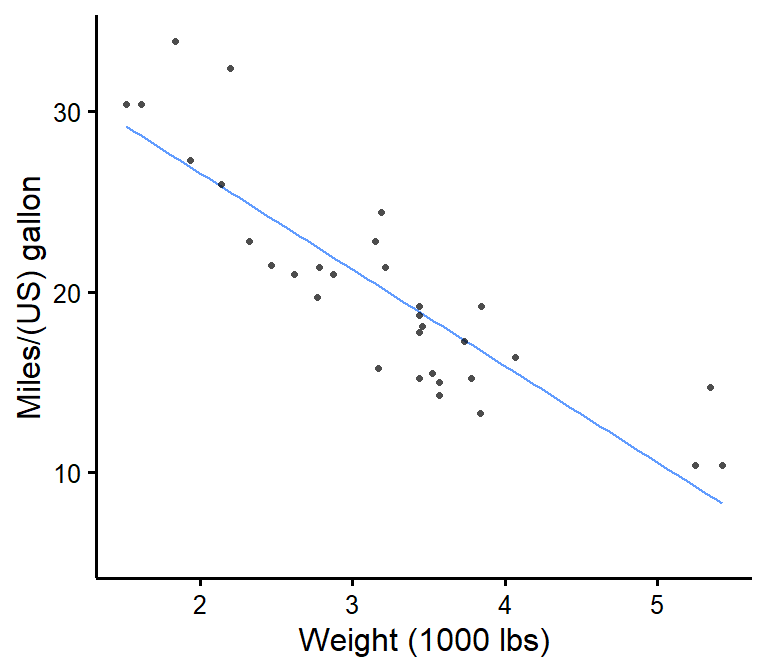### Have points “jittered”

Meaning randomly moved around a bit to prevent overplotting (when two or more points overlap, thus hiding information).

niceScatter(data = mtcars,
predictor = wt,
response = mpg,
has.jitter = TRUE)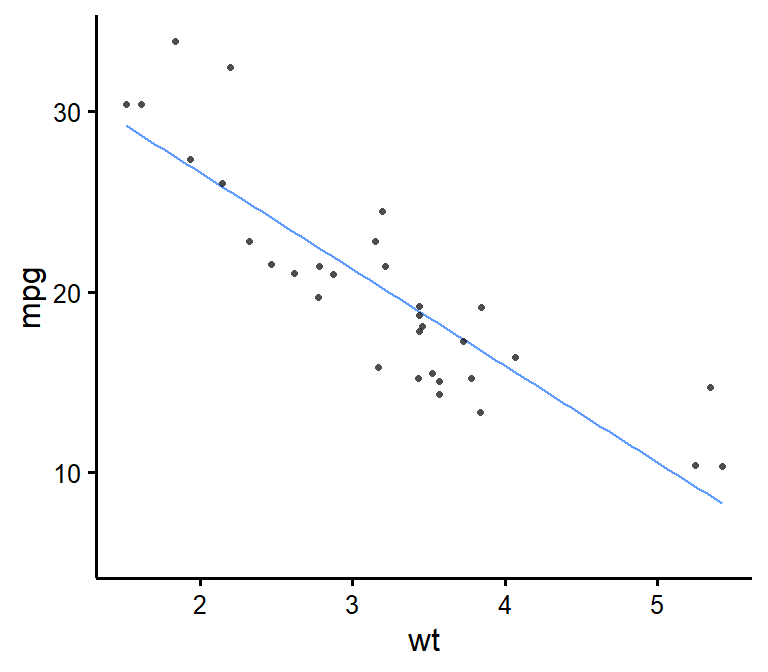### Change the transparency of the points

niceScatter(data = mtcars,
predictor = wt,
response = mpg,
alpha = 1) # default is 0.7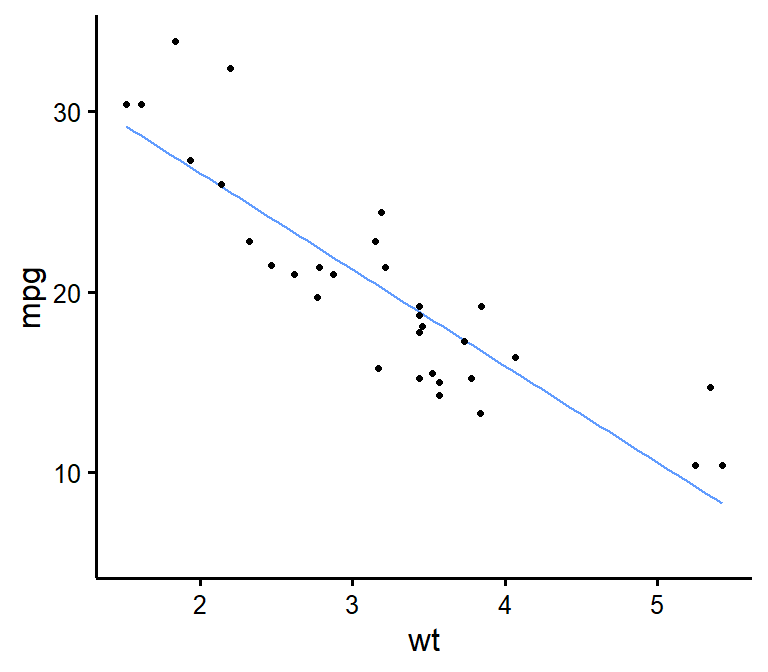### Remove points

niceScatter(data = mtcars,
predictor = wt,
response = mpg,
has.points = FALSE,
has.jitter = FALSE)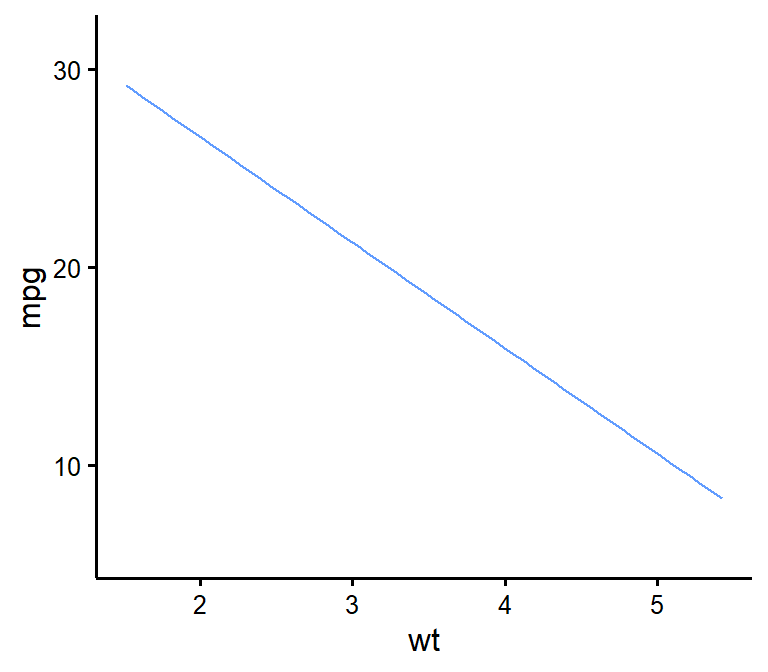niceScatter(data = mtcars,
predictor = wt,
response = mpg,
has.confband = TRUE)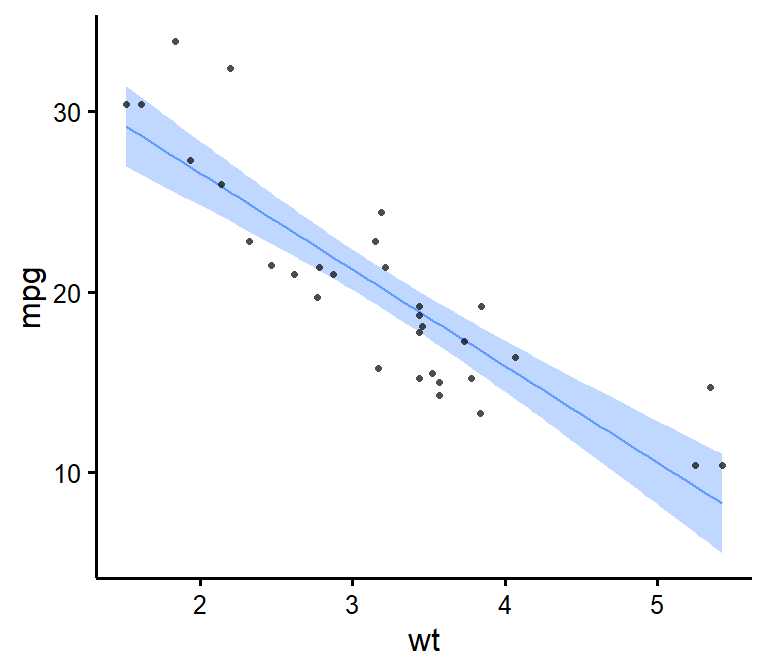### Set x- and y- scales manually

niceScatter(data = mtcars,
predictor = wt,
response = mpg,
xmin = 1,
xmax = 6,
xby = 1,
ymin = 10,
ymax = 35,
yby = 5)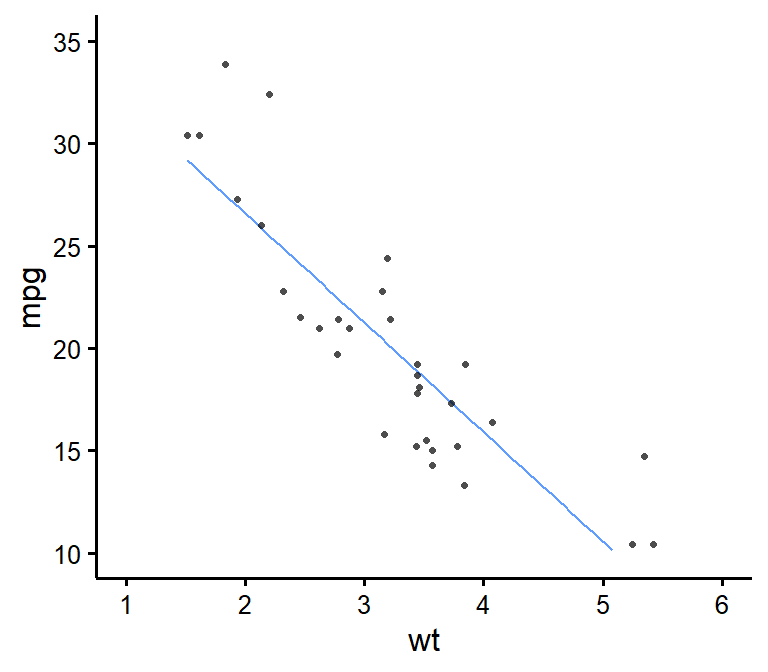### Change plot color

niceScatter(data = mtcars,
predictor = wt,
response = mpg,
colours = "blueviolet")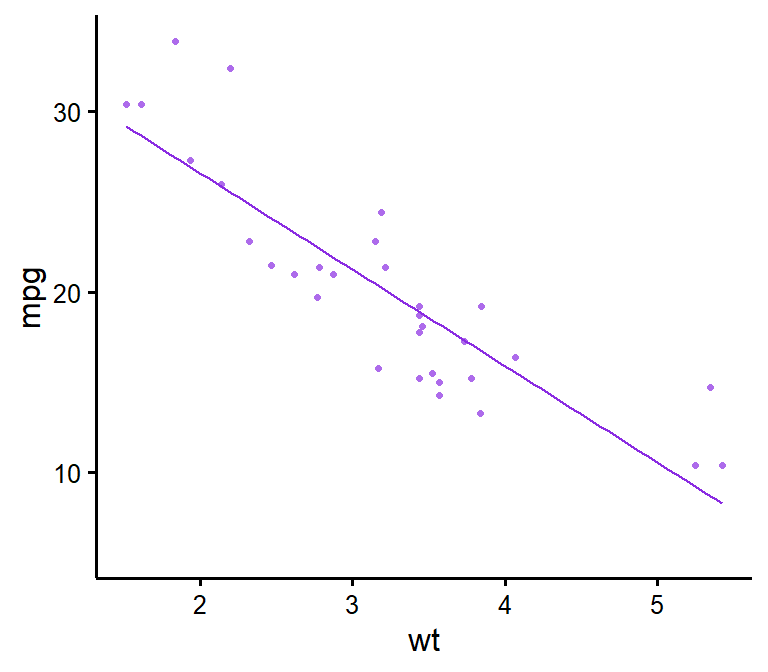### Add correlation coefficient to plot

niceScatter(data = mtcars,
predictor = wt,
response = mpg,
add.r = TRUE)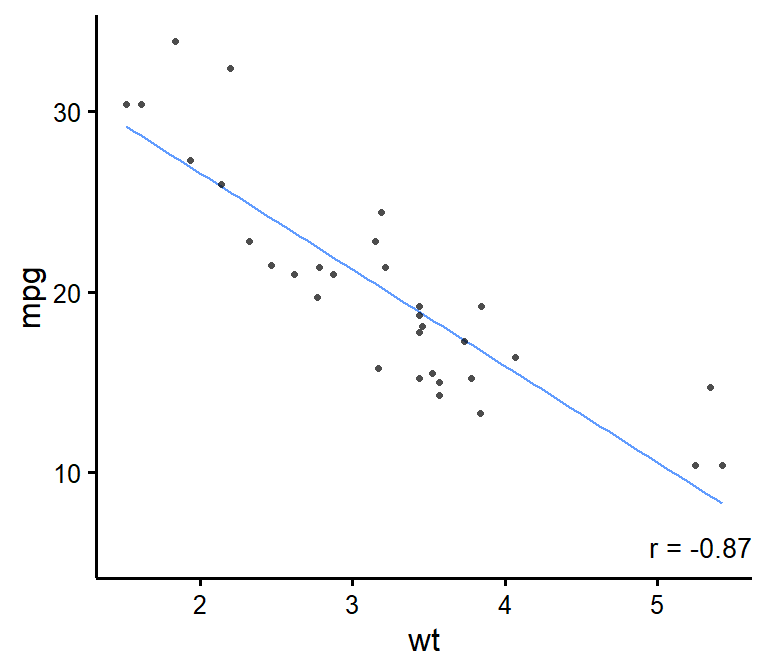### Change location of correlation coefficient

niceScatter(data = mtcars,
predictor = wt,
response = mpg,
r.x = 4,
r.y = 25)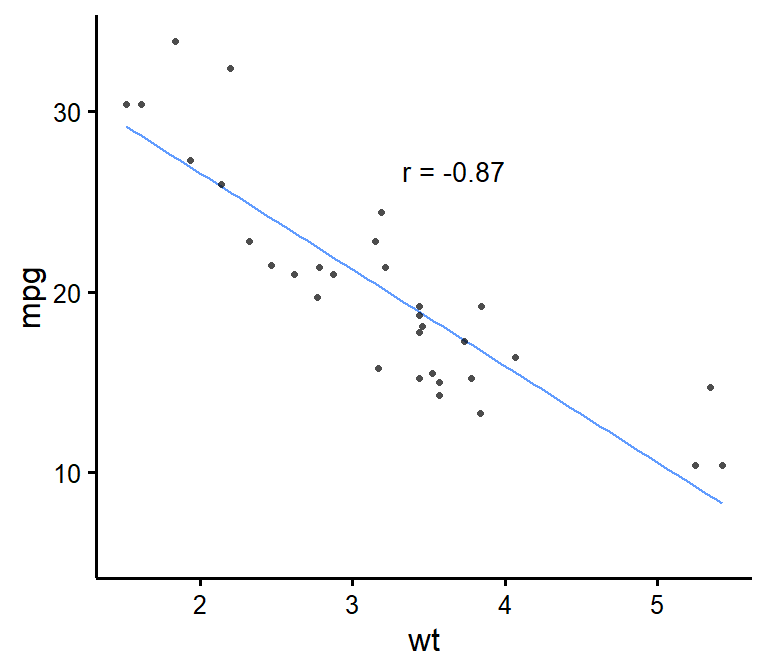### Plot by group

niceScatter(data = mtcars,
predictor = wt,
response = mpg,
group.variable = factor(mtcars$cyl)) # here we need to specify 'cyl' as a factor because it is numeric by default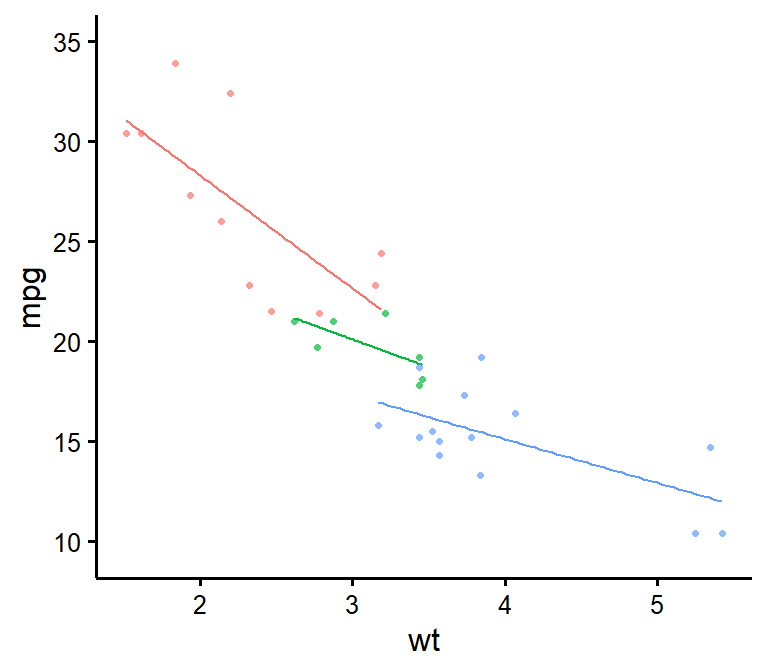### Use full range on the slope/confidence band niceScatter(data = mtcars, predictor = wt, response = mpg, group.variable = factor(mtcars$cyl),
has.fullrange = TRUE)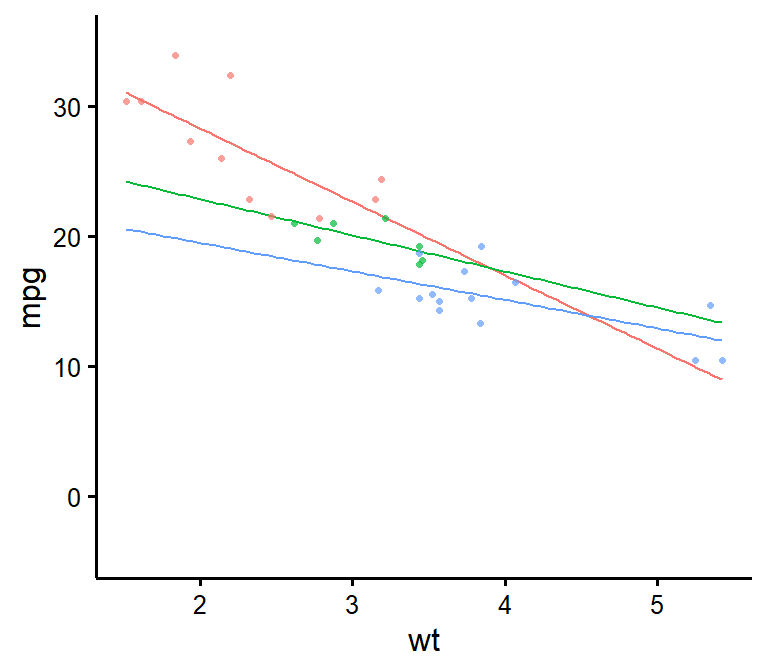niceScatter(data = mtcars,
predictor = wt,
response = mpg,
group.variable = factor(mtcars$cyl), has.legend = TRUE)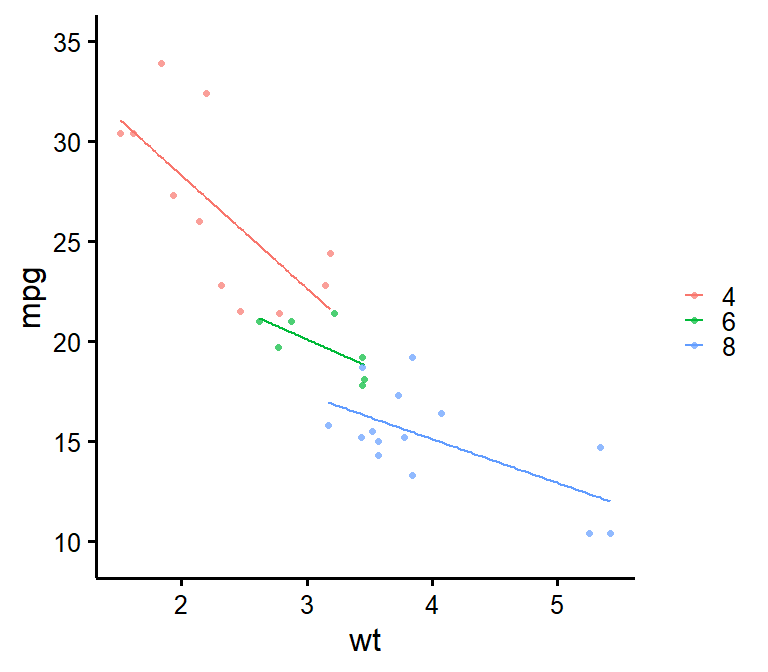### Change order of labels on the legend niceScatter(data = mtcars, predictor = wt, response = mpg, group.variable = factor(mtcars$cyl),
has.legend = TRUE,
groups.order = c(8,4,6)) # These are the levels of 'mtcars$cyl', so we place lvl 8 first, then lvl 4, etc.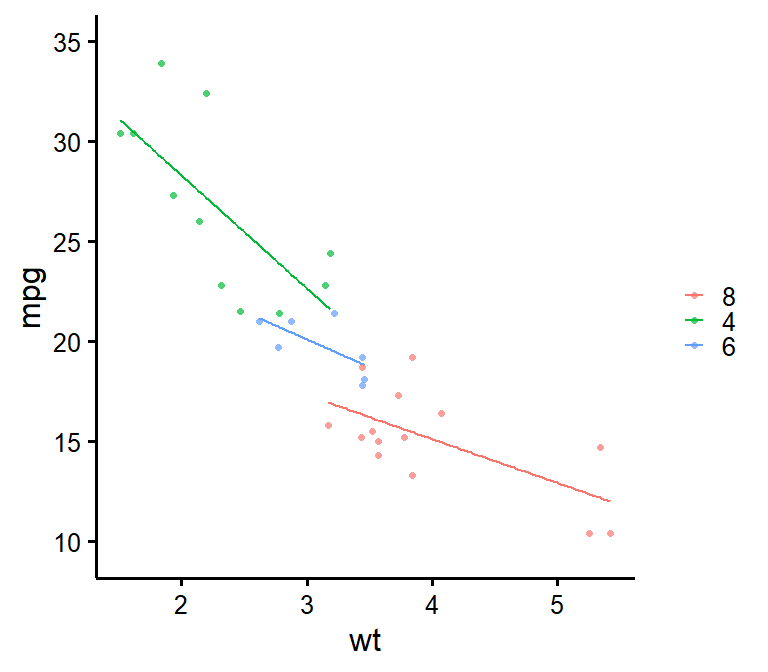### Change legend labels niceScatter(data = mtcars, predictor = wt, response = mpg, group.variable = factor(mtcars$cyl),
has.legend = TRUE,
groups.names = c("Weak","Average","Powerful")) # Warning: This applies after changing order of level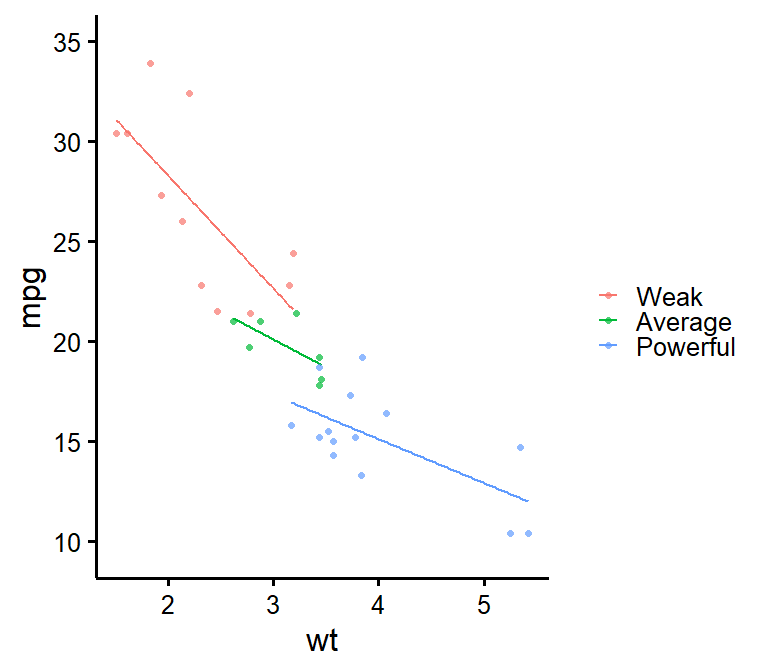**Warning**: This only changes labels and applies after changing order of level!
Always use groups.order first if you also need to use groups.names!
This is to make sure to have the right labels for the right groups!

### Add a title to legend

niceScatter(data = mtcars,
predictor = wt,
response = mpg,
group.variable = factor(mtcars$cyl), has.legend = TRUE, legend.title = "Cylinders")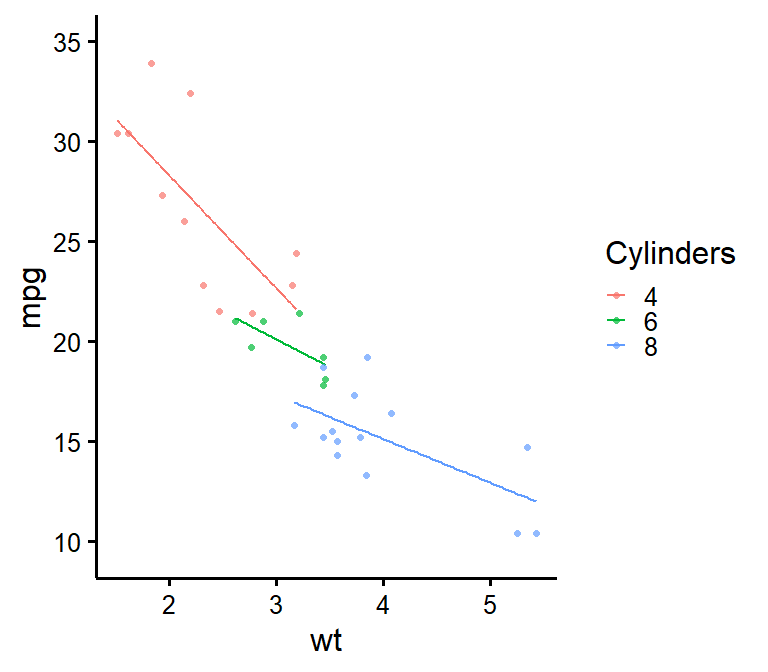### Plot by group + manually specify colours niceScatter(data = mtcars, predictor = wt, response = mpg, group.variable = factor(mtcars$cyl),
colours = c("burlywood","darkgoldenrod","chocolate"))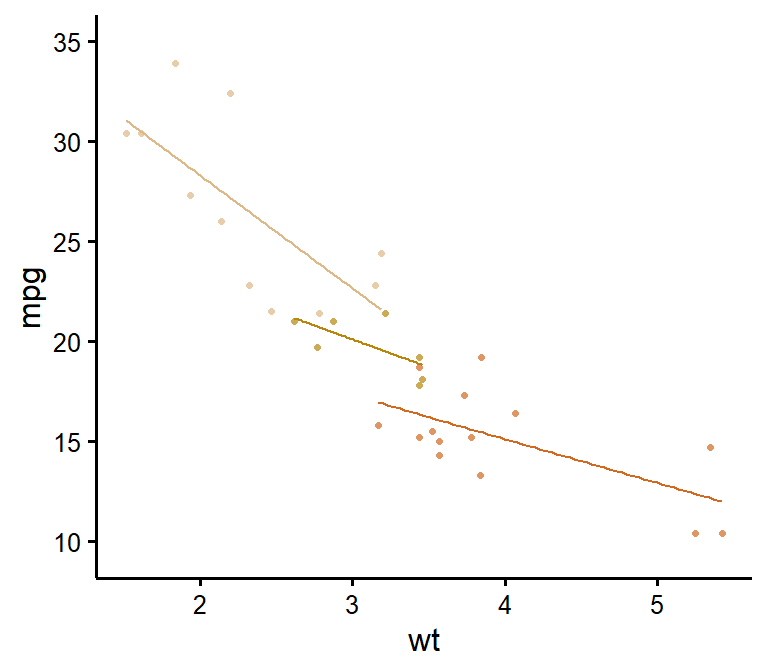### Plot by group + use different line types for each group

niceScatter(data = mtcars,
predictor = wt,
response = mpg,
group.variable = factor(mtcars$cyl), has.linetype = TRUE)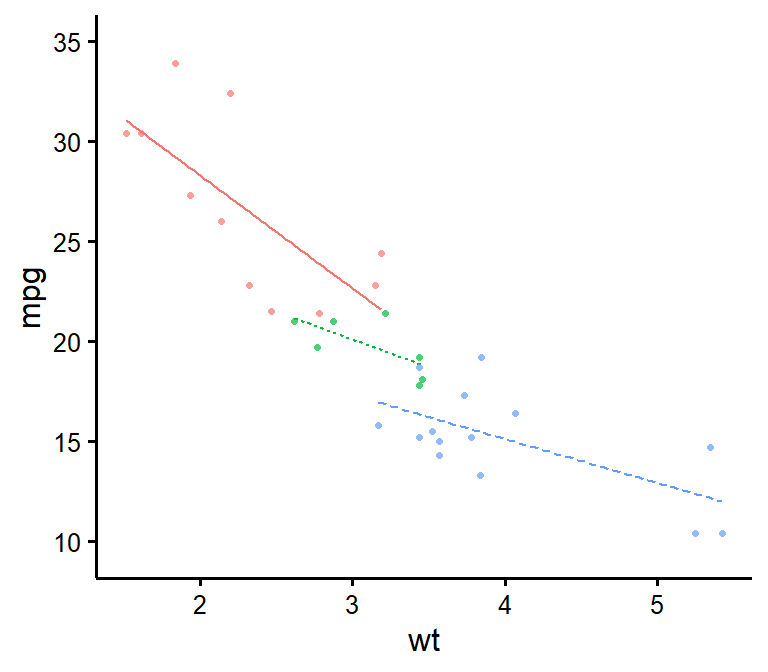### Plot by group + use different point shapes for each group niceScatter(data = mtcars, predictor = wt, response = mpg, group.variable = factor(mtcars$cyl),
has.shape = TRUE)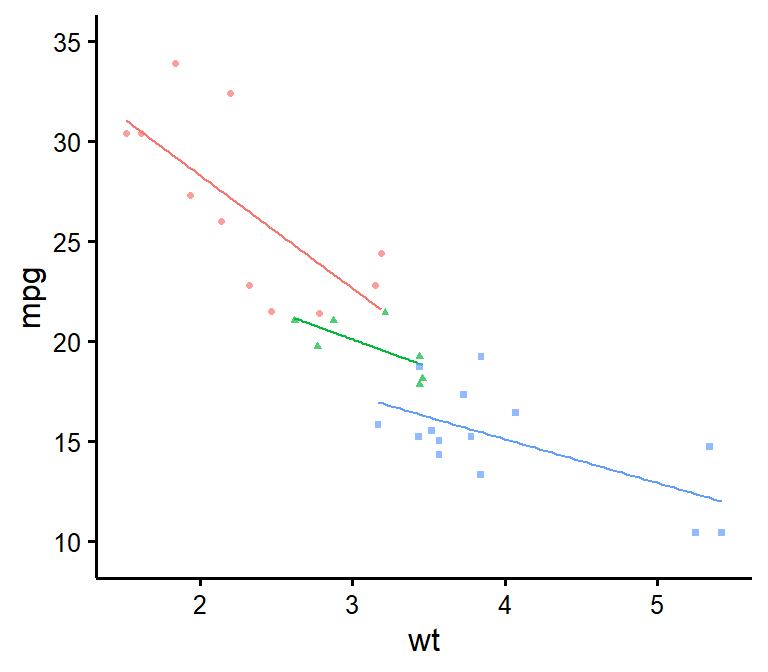### Plot by group, point shapes, line types, legend + no colours (black and white)

niceScatter(data = mtcars,
predictor = wt,
response = mpg,
group.variable = factor(mtcars$cyl), has.legend = TRUE, legend.title = "Cylinders", has.linetype = TRUE, has.shape = TRUE, colours = rep("black",3))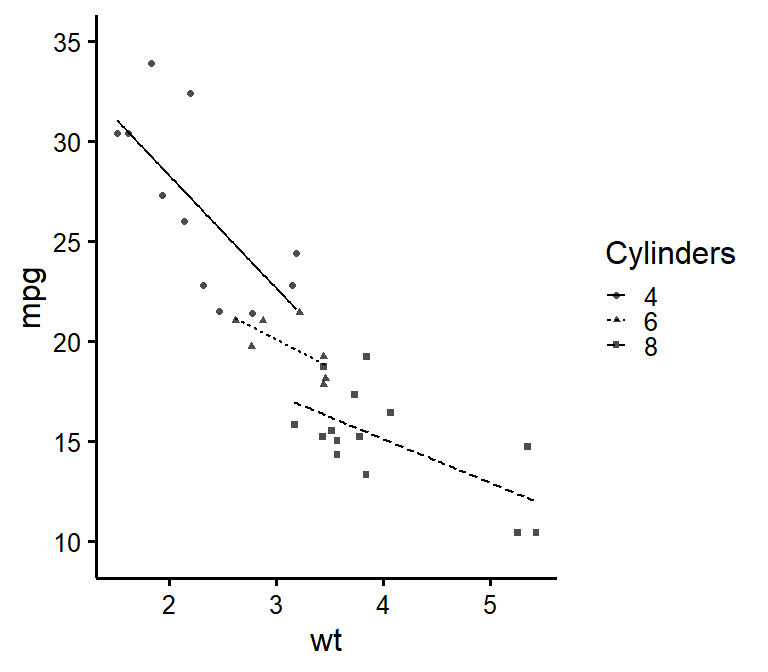### Putting it all together If you’d like to see all available options at once (a bit long): niceScatter(data = mtcars, predictor = wt, response = mpg, ytitle = "Miles/(US) gallon", xtitle = "Weight (1000 lbs)", has.points = FALSE, has.jitter = TRUE, alpha = 1, has.confband = TRUE, has.fullrange = FALSE, group.variable = factor(mtcars$cyl),
has.linetype = TRUE,
has.shape = TRUE,
xmin = 1,
xmax = 6,
xby = 1,
ymin = 10,
ymax = 35,
yby = 5,
r.x = 5.5,
r.y = 25,
colours = c("burlywood","darkgoldenrod","chocolate"),
has.legend = TRUE,
legend.title = "Cylinders",
groups.names = c("Weak","Average","Powerful"))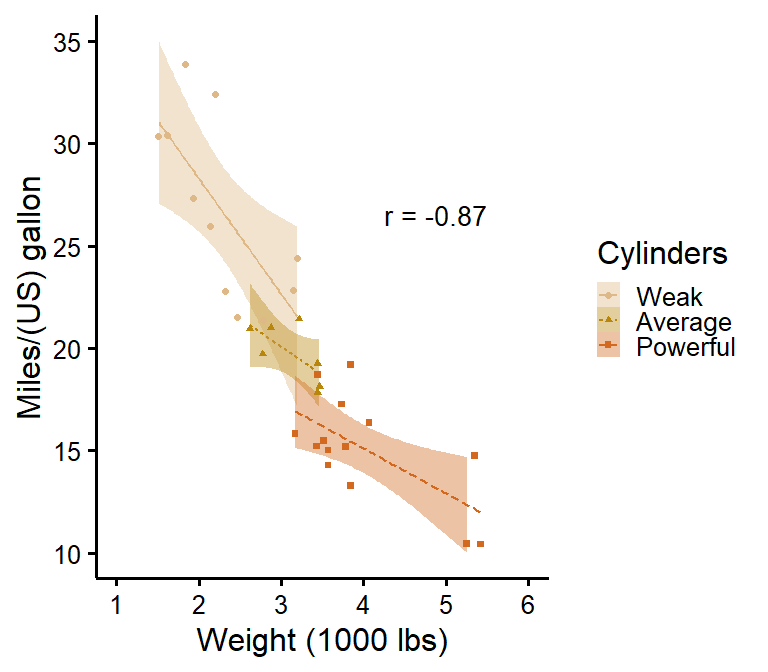## Special situation: Add group average

There’s no straightforward way to add group average, so here’s a hack to do it. We first have to create a second data set with another “group” that will be used as the average.

new.Data <- mtcars # This simply copies the 'mtcars' dataset
new.Data$cyl <- "Average" # That would be your "Group" variable normally # And this operation fills all cells of that column with the word "Average" to identify our new 'group' XData <- rbind(mtcars,new.Data) # This adds the new "Average" group rows to the original data rows Then we need to create a FIRST layer of just the slopes. We add transparency to the group lines except the group average to emphasize the group average (with the new argument manual.slope.alpha). (p <- niceScatter(data = XData, predictor = wt, response = mpg, has.points = FALSE, has.legend = TRUE, group.variable = XData$cyl,
colours = c("black", "#00BA38", "#619CFF", "#F8766D"), # We add colours manually because we want average to be black to stand out
groups.order = c("Average","4","6","8"), # We do this to have average on top since it's the most important
manual.slope.alpha = c(1,0.5,0.5,0.5))) # This adds 50% transparency to all lines except the first one (Average) which is 100%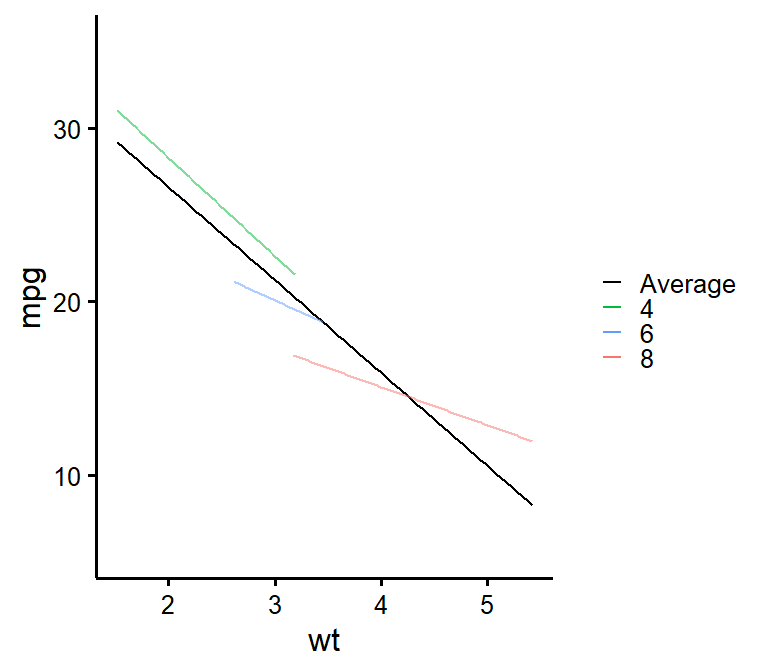Finally we are ready to add a SECOND layer of just the points on top of our previous layer. We use standard ggplot syntax for this.

p + geom_point(data = mtcars,
size = 2,
alpha = 0.5,
shape = 16, # We use shape 16 because the default shape 19 sometimes causes problems when exporting to PDF
mapping = aes(x = wt,
y = mpg,
colour = factor(cyl),
fill = factor(cyl)))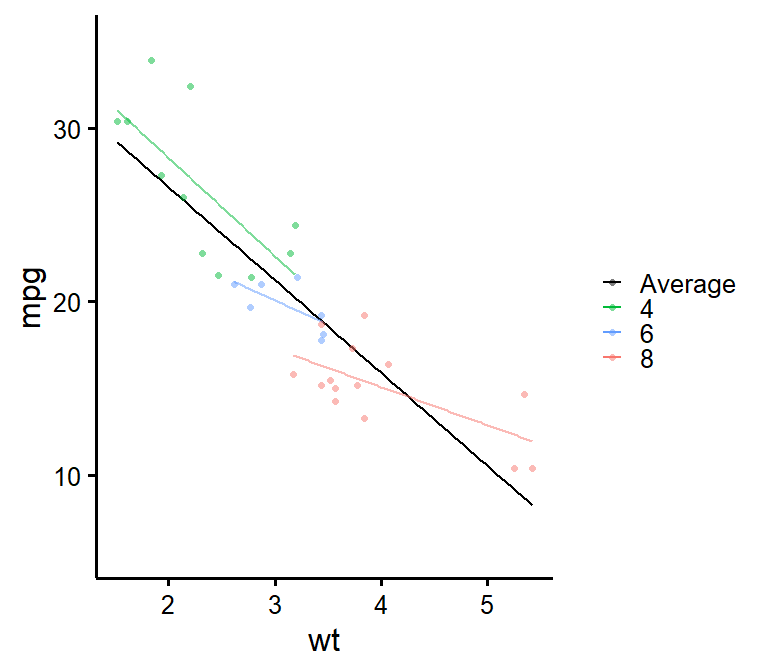If you’d like instead to still show the group points but only the black average line, you can do the following as first layer:

(p <- niceScatter(data = mtcars,
predictor = wt,
response = mpg,
has.points = FALSE,
has.legend = TRUE, # This argument is important else the next legend won't appear on the second layer!
colours = "black"))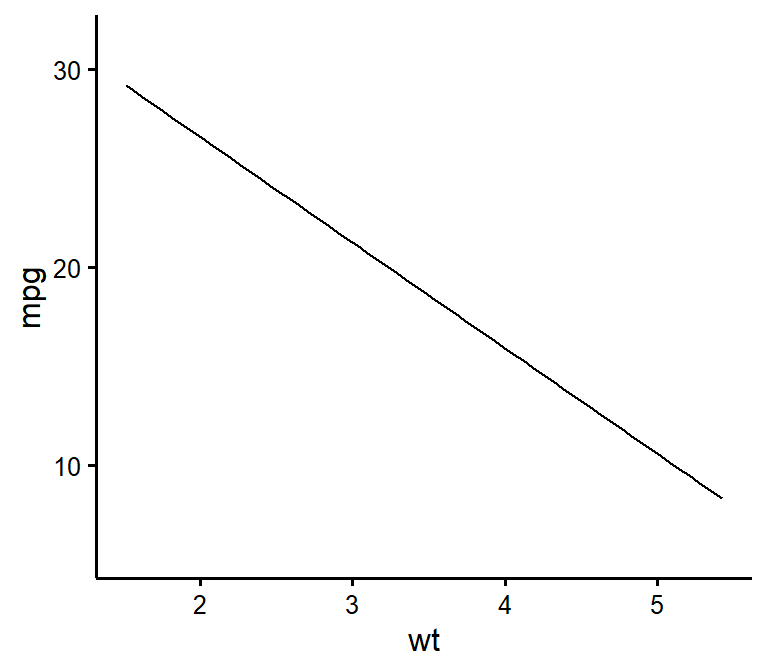Then to add the points as second layer we do the same as before:

p + geom_point(data = mtcars,
size = 2,
alpha = 0.5,
shape = 16,
mapping = aes(x = wt,
y = mpg,
colour = factor(cyl)))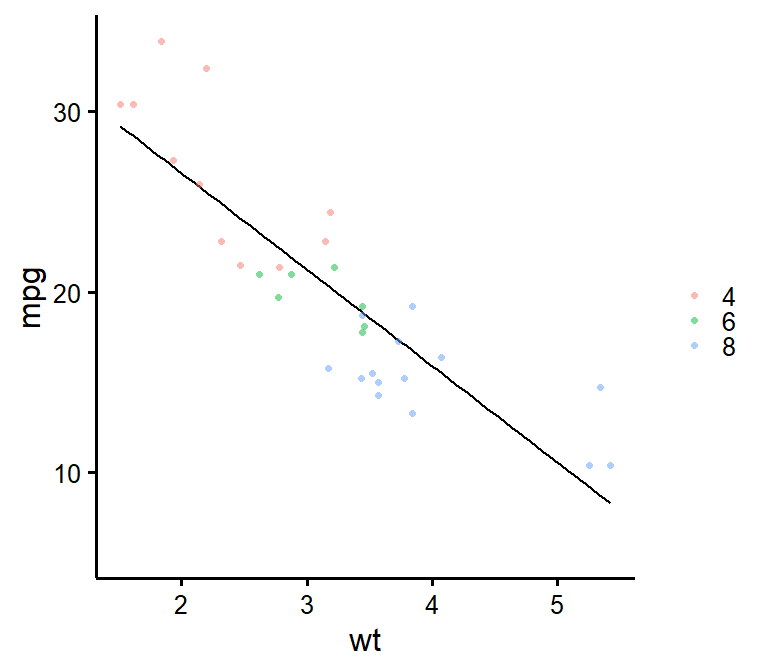$$~$$

$$~$$

### Concluding Statement

Make sure to check out this page again if you use the code after a time or if you encounter errors, as I periodically update or improve the code.

You can always edit the function to suit your purposes, or contact me for questions or requests to modify this function at remitheriault.wixsite.com/site/contact! Thanks for reading my guide! :) $$~$$

$$~$$

$$~$$

$$~$$

$$~$$

Updated 2020-09-17 (added: argument add.r)

$$~$$

$$~$$

$$~$$

$$~$$

$$~$$

$$~$$